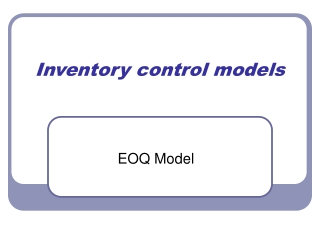DownloadDownload PresentationInventory control models

# Inventory control models

Télécharger la présentation## Inventory control models

- - - - - - - - - - - - - - - - - - - - - - - - - - - E N D - - - - - - - - - - - - - - - - - - - - - - - - - - -
##### Presentation Transcript

1. Inventory control models EOQ Model

2. Learning objective • After this class the students should be able to: • calculate the order quantity that minimize the total cost inventory, based on the EOQ model and • analyze the implication of this model.

3. Time management • The expected time to deliver this module is 50 minutes. 30 minutes are reserved for team practices and exercises and 20 minutes for lecture.

4. Inventory & inventory system • Inventory is the set of items that an organization holds for later use by the organization. An inventory system is a set of policies that monitors and controls inventory. It determines how much of each item should be kept, when low items should be replenished, and how many items should be ordered or made when replenishment is needed.

5. Basic types of inventory • independent demand, • dependent demand, and • supplies.

6. Independent Demand • Independent demanditems are those items that we sell to customers. • Dependent demanditems are those items whose demand is determined by other items. Demand for a car translates into demand for four tires, one engine, one transmission, and so on. The items used in the production of that car (the independent demand item) are the dependent demand items. • Supplies are items such as copier paper, cleaning materials, and pens that are not used directly in the production of independent demand items

7. Why hold Inventory • Each team is invited to discuss for 10 minutes about what they figure out to be the reasons that the organizations maintain inventory. At the and, they have to present a list of their supposed reasons.

8. Five reasons • To decouple workcenters; • To meet variations in demand; • To allow flexible production schedules; • As a safeguard against variations in delivery time; and • To get a lower price.

9. The cost of Inventory • Holding costs; • Setup costs; • Ordering costs; and • Shortage costs.

10. The Economic Order Quantity Model • Assumptions: • 1. Production is instantaneous.There is no capacity constraint and the entire lot is produced simultaneously. • 2. Delivery is immediate.There is no time lag between production and availability to satisfy demand. • 3. Demand is deterministic.There is no uncertainty about the quantity or timing of demand. • 4. Demand is constant over time. In fact, it can be represented as a straight line, so that if annual demand is 365 units this translates into a daily demand of one unit. • 5 A production run incurs a constant setup cost. Regardless of the size of the lot or the status of the factory, the setup cost is the same. • 6. Products can be analyzed singly.Either there is only a single product or conditions exist that ensure reparability of products.

11. Notation • D= Demand rate (in units per year). • c = Unit production cost, not counting setup or inventory costs (in dollars per unit). • A = Constant setup (ordering) cost to produce (purchase) a lot (in dollars). • h = Holding cost • Q = Lot size (in units); this is the decision variable

12. The model Inventory versus time in the EOQ model

13. The model • average inventory level: • The holding cost per unit: • The setup costper unit: • The production cost per unit:

14. Economic order quantity

15. Economic order quantity

16. Exercise Each is invited to analyze the following insights, based on the EOQ model (20 minutes): • “There is a tradeoff between lot size and inventory • “Holding and setup cost are fairly insensitive to lot size”

17. What-if

18. Open excel file What-if The minimum cost obtained by using the economic order quantity is \$952.50, so increasing the order quantity by 10% leads a total cost increase of only \$4.30. Changing the order quantity by a small amount has very little effect on the cost, because EOQ formula gives robust solutions.

19. Reference • Factory Physics. Hopp & Spearman, Irvin, 1996, Chapter 2